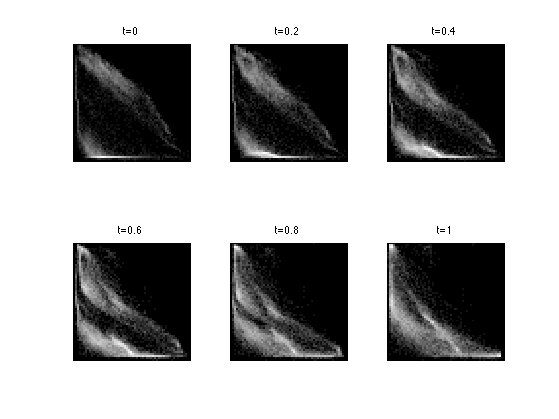$\newcommand{\NN}{\mathbb{N}} \newcommand{\CC}{\mathbb{C}} \newcommand{\GG}{\mathbb{G}} \newcommand{\LL}{\mathbb{L}} \newcommand{\PP}{\mathbb{P}} \newcommand{\QQ}{\mathbb{Q}} \newcommand{\RR}{\mathbb{R}} \newcommand{\VV}{\mathbb{V}} \newcommand{\ZZ}{\mathbb{Z}} \newcommand{\FF}{\mathbb{F}} \newcommand{\KK}{\mathbb{K}} \newcommand{\UU}{\mathbb{U}} \newcommand{\EE}{\mathbb{E}} \newcommand{\Aa}{\mathcal{A}} \newcommand{\Bb}{\mathcal{B}} \newcommand{\Cc}{\mathcal{C}} \newcommand{\Dd}{\mathcal{D}} \newcommand{\Ee}{\mathcal{E}} \newcommand{\Ff}{\mathcal{F}} \newcommand{\Gg}{\mathcal{G}} \newcommand{\Hh}{\mathcal{H}} \newcommand{\Ii}{\mathcal{I}} \newcommand{\Jj}{\mathcal{J}} \newcommand{\Kk}{\mathcal{K}} \newcommand{\Ll}{\mathcal{L}} \newcommand{\Mm}{\mathcal{M}} \newcommand{\Nn}{\mathcal{N}} \newcommand{\Oo}{\mathcal{O}} \newcommand{\Pp}{\mathcal{P}} \newcommand{\Qq}{\mathcal{Q}} \newcommand{\Rr}{\mathcal{R}} \newcommand{\Ss}{\mathcal{S}} \newcommand{\Tt}{\mathcal{T}} \newcommand{\Uu}{\mathcal{U}} \newcommand{\Vv}{\mathcal{V}} \newcommand{\Ww}{\mathcal{W}} \newcommand{\Xx}{\mathcal{X}} \newcommand{\Yy}{\mathcal{Y}} \newcommand{\Zz}{\mathcal{Z}} \newcommand{\al}{\alpha} \newcommand{\la}{\lambda} \newcommand{\ga}{\gamma} \newcommand{\Ga}{\Gamma} \newcommand{\La}{\Lambda} \newcommand{\Si}{\Sigma} \newcommand{\si}{\sigma} \newcommand{\be}{\beta} \newcommand{\de}{\delta} \newcommand{\De}{\Delta} \renewcommand{\phi}{\varphi} \renewcommand{\th}{\theta} \newcommand{\om}{\omega} \newcommand{\Om}{\Omega} \renewcommand{\epsilon}{\varepsilon} \newcommand{\Calpha}{\mathrm{C}^\al} \newcommand{\Cbeta}{\mathrm{C}^\be} \newcommand{\Cal}{\text{C}^\al} \newcommand{\Cdeux}{\text{C}^{2}} \newcommand{\Cun}{\text{C}^{1}} \newcommand{\Calt}{\text{C}^{#1}} \newcommand{\lun}{\ell^1} \newcommand{\ldeux}{\ell^2} \newcommand{\linf}{\ell^\infty} \newcommand{\ldeuxj}{{\ldeux_j}} \newcommand{\Lun}{\text{\upshape L}^1} \newcommand{\Ldeux}{\text{\upshape L}^2} \newcommand{\Lp}{\text{\upshape L}^p} \newcommand{\Lq}{\text{\upshape L}^q} \newcommand{\Linf}{\text{\upshape L}^\infty} \newcommand{\lzero}{\ell^0} \newcommand{\lp}{\ell^p} \renewcommand{\d}{\ins{d}} \newcommand{\Grad}{\text{Grad}} \newcommand{\grad}{\text{grad}} \renewcommand{\div}{\text{div}} \newcommand{\diag}{\text{diag}} \newcommand{\pd}{ \frac{ \partial #1}{\partial #2} } \newcommand{\pdd}{ \frac{ \partial^2 #1}{\partial #2^2} } \newcommand{\dotp}{\langle #1,\,#2\rangle} \newcommand{\norm}{|\!| #1 |\!|} \newcommand{\normi}{\norm{#1}_{\infty}} \newcommand{\normu}{\norm{#1}_{1}} \newcommand{\normz}{\norm{#1}_{0}} \newcommand{\abs}{\vert #1 \vert} \newcommand{\argmin}{\text{argmin}} \newcommand{\argmax}{\text{argmax}} \newcommand{\uargmin}{\underset{#1}{\argmin}\;} \newcommand{\uargmax}{\underset{#1}{\argmax}\;} \newcommand{\umin}{\underset{#1}{\min}\;} \newcommand{\umax}{\underset{#1}{\max}\;} \newcommand{\pa}{\left( #1 \right)} \newcommand{\choice}{ \left\{ \begin{array}{l} #1 \end{array} \right. } \newcommand{\enscond}{ \left\{ #1 \;:\; #2 \right\} } \newcommand{\qandq}{ \quad \text{and} \quad } \newcommand{\qqandqq}{ \qquad \text{and} \qquad } \newcommand{\qifq}{ \quad \text{if} \quad } \newcommand{\qqifqq}{ \qquad \text{if} \qquad } \newcommand{\qwhereq}{ \quad \text{where} \quad } \newcommand{\qqwhereqq}{ \qquad \text{where} \qquad } \newcommand{\qwithq}{ \quad \text{with} \quad } \newcommand{\qqwithqq}{ \qquad \text{with} \qquad } \newcommand{\qforq}{ \quad \text{for} \quad } \newcommand{\qqforqq}{ \qquad \text{for} \qquad } \newcommand{\qqsinceqq}{ \qquad \text{since} \qquad } \newcommand{\qsinceq}{ \quad \text{since} \quad } \newcommand{\qarrq}{\quad\Longrightarrow\quad} \newcommand{\qqarrqq}{\quad\Longrightarrow\quad} \newcommand{\qiffq}{\quad\Longleftrightarrow\quad} \newcommand{\qqiffqq}{\qquad\Longleftrightarrow\qquad} \newcommand{\qsubjq}{ \quad \text{subject to} \quad } \newcommand{\qqsubjqq}{ \qquad \text{subject to} \qquad }$

Sliced Optimal Transport

# Sliced Optimal Transport

This tour explore the used of the sliced Wasserstein distance to approximate optimal transport.

## Installing toolboxes and setting up the path.

You need to download the following files: signal toolbox and general toolbox.

You need to unzip these toolboxes in your working directory, so that you have toolbox_signal and toolbox_general in your directory.

For Scilab user: you must replace the Matlab comment '%' by its Scilab counterpart '//'.

Recommandation: You should create a text file named for instance numericaltour.sce (in Scilab) or numericaltour.m (in Matlab) to write all the Scilab/Matlab command you want to execute. Then, simply run exec('numericaltour.sce'); (in Scilab) or numericaltour; (in Matlab) to run the commands.

Execute this line only if you are using Matlab.

getd = @(p)path(p,path); % scilab users must *not* execute this


Then you can add the toolboxes to the path.

getd('toolbox_signal/');
getd('toolbox_general/');


## Wasserstein Distance

We consider data $$f \in \RR^{N \times d}$$, that corresponds for instance to an image of $$N$$ pixels, with $$d=1$$ for grayscale image and $$d=3$$ for color image. We denote $$f = (f_i)_{i=1}^N$$ with $$f_i \in \RR^d$$ the elements of the data.

The discrete (empirical) distribution in $$\RR^d$$ associated to this data $$f$$ is the sum of Diracs $\mu_f = \frac{1}{N} \sum_{i=1}^N \de_{f_i}.$

An optimal assignement between two such vectors $$f,g \in \RR^{N \times d}$$ is a permutation $$\si \in \Si_N$$ that minimizes $\si^\star \in \uargmin{\si \in \Si_N} \sum_{i=1}^N \norm{f_i - g_{\si(i)}}^2.$

This optimal assignement defines the $$L^2$$ Wasserstein distance between the associated point clouds distributions $W_2(\mu_f,\mu_g)^2 = \sum_{i=1}^N \norm{f_i - g_{\si(i)}}^2 = \norm{f - g \circ \si}_2^2$ where $$g \circ \si = (g_{\si(i)})_i$$ is the re-ordered points cloud.

## 1-D Optimal Transport

Unfortunately, computing $$\si^\star$$ when $$d>1$$ is computationaly more difficult. Best known algorithms (e.g. Hungarian algorithm or linear programming) have a running time of roughly $$O(N^3)$$.

For 1-D data, $$d=1$$, one can compute explicitely an optimal assignement $$\si^\star \in \Si_N$$ for any cost $$C(u,v) = \phi(\abs{u-v})$$ where $$\phi : \RR \rightarrow \RR$$ is a convex function. This is thus the case for the $$L^p$$ optimal transport.

This is obtained by computing two permutations $$\si_f, \si_g \in \Si_N$$ that order the values of the data $f_{\si_f(1)} \leq f_{\si_f(2)} \leq \ldots f_{\si_f(N)}$ $g_{\si_g(1)} \leq g_{\si_g(2)} \leq \ldots g_{\si_g(N)}.$

An optimal assignement is then optained by assigning, for each $$k$$, the index $$i = \si_f(k)$$ to the index $$\si^\star(i) = \si_g(k)$$, i.e. $\si^\star = \si_g \circ \si_f^{-1}$ where $$\si_f^{-1}$$ is the inverse permutation, that satisfies $\si_f^{-1} \circ \si_f = \text{Id}$.

## Wasserstein Projection

Given two point clouds $$f,g \in \RR^{N \times d}$$, we define $$\Pi_g(f)$$ as the orthogonal projection of $$f$$ on the set $\Hh_g = \enscond{m \in \RR^N}{ \mu_m = \mu_g }.$ This projection is actually equal to $\Pi_g(f) = g \circ \si^\star = (g_{\si^\star(i)})_{i=1}^N,$ where $$\si^\star$$ is the optimal assignement beteen $$f$$ and $$g$$.

For 1-D point clouds ($$d=1$$), since computing $$\si^\star$$ is easy, one can compute easily this projection.

We define the operator $$P(f,g)$$ such that for for 1-D point clouds, $$f,g \in \RR^N$$ $$P(f,g) = \Pi_g(f)$$ is the orthogonal projection of $$f$$ onto the histogram constraints $$\Hh_g$$.

options.rows=1;
P = @(f,g)perform_hist_eq(f,g,options);


This operator operates over 1-D distributions, and is extended to higher dimensions by applying 1-D projection independently along each dimension. Important: note that when $$d>1$$, one does not have in general $$P(f,g) = \Pi_g(f)$$.

## Sliced Wasserstein Distance

To obtain approximated assignements for large scale imaging problems, we introduce the following sliced approximation $SW_2(\mu_f,\mu_g)^2 = \int_{\mathbb{S}^{d-1}} W_2( \mu_{f_\th}, \mu_{g_\th} )^2 \, d \th$ which integrate on the $$d$$-dimensional sphere $\mathbb{S}^{d-1} = \enscond{\th \in \RR^d}{ \norm{\th}=1 }$ the 1-D Wasserstein distance $$W_2( \mu_{f_\th}, \mu_{g_\th} )$$ between 1-D projected point clouds $f_\th = ( \dotp{f_i}{\th} )_{i=1}^N \in \RR^N.$

Following an idea initially proposed by Marc Bernot, this sliced distance is introduced in:

J. Rabin, G. Peyre, J. Delon, M. Bernot, Wassertein Barycenter and its Applications to Texture Mixing Proc. SSVM'11, Springer, LNCS vol. 6667, pp. 435-446, 2011.

## Sliced Wasserstein Projection

We would like to compute the orthogonal projection $$\tilde f = \Pi_g(f)$$ on the statistical constraint $$\Hh_g$$. This is computationally intractable for large scala datasets, since it requires to compute the optimal assignement $$\si^\star$$ between $$f$$ and $$g$$.

We approximate $$\tilde f$$ by computing a new dataset that is both a local minimizer of the sliced Wasserstein distance to $$\mu_g$$ $E(m) = W_2(\mu_m,\mu_g)$ and that is close to $$f$$.

One can show that $$E$$ is a $$C^1$$ functional. In order to obtain a cloud that is close to $$f$$, we start a gradient descent of $$E$$ starting from $$f$$ $f^{(0)}=f \qandq f^{(\ell+1)} = f^{(\ell)} - \tau_\ell \nabla E(f^{(\ell)})$ for some step size $$\tau_\ell>0$$.

This gradient descent is still untractable to compute because computing $$\nabla E$$ requires an integration on the whole sphere $$\mathbb{S}^{d-1}$$. We thus use instead a stochastic gradient descent method. At each iteration, we compute at random an orthogonal basis $$\Theta = (\th_1,\ldots,\th_\ell)$$ of $$\RR^d$$. We update the current iterates by minimzing a partial sliced distance $f^{(\ell+1)} = f^{(\ell)} - \tau_\ell \nabla E_\Theta(f^{(\ell)})$ where the integral in the definition of the distance is replaced by a discrete sum $E_\Theta(m) = \sum_{k=1}^d W_2( \mu_{m_{\th_k}}, \mu_{g_{\th_k}} )^2.$

One can show that computing this stochastic gradient step is equivalent to computing $f^{(\ell+1)} = (1-\tau_\ell) f^{(\ell)} + \tau_\ell \tilde f^{(\ell)}$ where $$\tilde f^{(\ell)}$$ is obtained by equalizing independantly each coordinate of $$f^{(\ell)}$$ and $$g$$ in the orthobasis $$\Theta$$. This corresponds to use the coordinate-wise projector $$P$$ $\tilde f^{(\ell)} = \Theta P( \Theta^* f^{(\ell)}, \Theta^* g )$ where $$\Theta^*$$ is the transpose operator, i.e. $$\Theta^*(u) = (\dotp{u}{\theta_k})_{k=1}^d$$

We show numerically that this gradient descent scheme converges to a point that is within the statistical constraint, i.e. $f^{(\ell)} \overset{\ell \rightarrow +\infty}{\longrightarrow} \tilde f \qwhereq \mu_{\tilde f} = \mu_g.$ This means that at convergence, one obtains an assignement between $$f$$ and $$g$$, i.e. $$\tilde f = g \circ \si$$ for some $$\si$$. It is important to note that in general, this $$\si$$ is not the optimal assignement $$\si^\star$$, although we oberse numerically that it is close to be optimal for many large scale imaging problems.

## Matching of 2-D Distributions

We apply this stochastic gradient descent to perform the matching of a pair of 2-D distributions, hence $$d=2$$.

Number of points.

N = 300;


Dimension of the clouds.

d = 2;


First distribution of points, inside a square.

f = rand(2,N)-.5;


Second distribution of points, inside an anulus.

theta = 2*pi*rand(1,N);
r = .8 + .2*rand(1,N);
g = [cos(theta).*r; sin(theta).*r];


Shortcut for displaying point clouds.

plotp = @(x,col)plot(x(1,:)', x(2,:)', 'o', 'MarkerSize', 10, 'MarkerEdgeColor', 'k', 'MarkerFaceColor', col, 'LineWidth', 2);


Display.

clf; hold on;
plotp(f, 'b');
plotp(g, 'r');
axis('off'); axis('equal');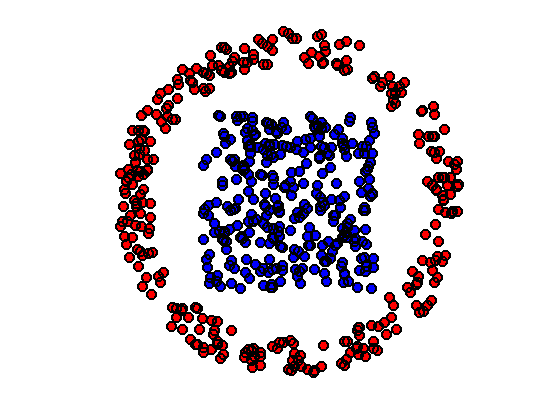We use a fixed gradient step size $$\tau_\ell=\tau$$. While stochastic gradient descent require in theory a decaying step size, it appears numerically that a fixed step size works well to minimize SW.

tau = .2;


Intialize $$f^{(0)}=f$$.

f1 = f;


Compute an orthogonal coordinate system for this iteration.

[Theta,~] = qr(randn(d));


Perform the gradient step update.

f1 = (1-tau)*f1 + tau * Theta * P(Theta'*f1, Theta'*g);


Display $$f$$ and $$g$$, together with the position $$f^{(1)}$$ at the first iteration (in yellow).

clf; hold on;
plotp(f, 'b');
plotp(g, 'r');
plotp(f1, 'y');
axis('off'); axis('equal');Exercice 1: (check the solution) Iterate many time the randomized assignement until convergence of $$\tilde f$$. The random projector $$\Theta$$ should be re-computed at each iteration.

exo1;Display final configuration with the matching.

clf;
hold on;
h = plot([f(1,:);f1(1,:)], [f(2,:);f1(2,:)], 'k');
set(h, 'LineWidth', 2);
plotp(f, 'b');
plotp(g, 'r');
axis('off'); axis('equal');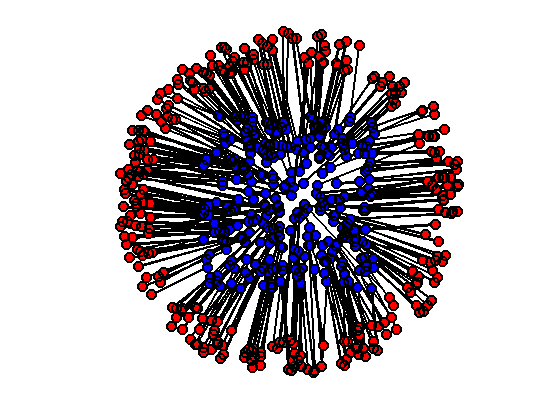An average point cloud is obtained by interpolation $\forall t \in [0,1], \quad f_t = (1-t) f + t \tilde f.$

t = .5;
ft = (1-t)*f + t*f1;


Display the barycenter in magenta for $$t=1/2$$ (midway point cloud).

clf;
hold on;
plotp(f, 'b');
plotp(g, 'r');
plotp(ft, [t 0 1-t]);
axis('off'); axis('equal');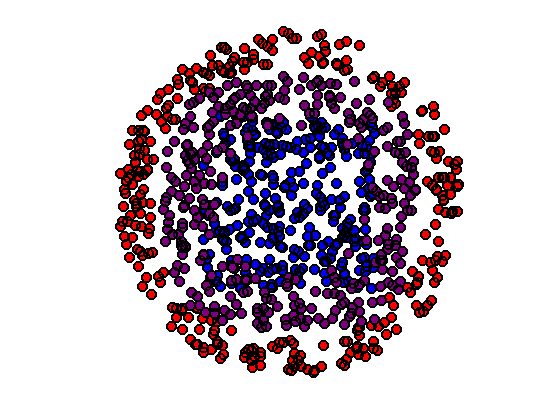Exercice 2: (check the solution) Show the progressive interpolation for varying $$t \in [0,1]$$.

exo2;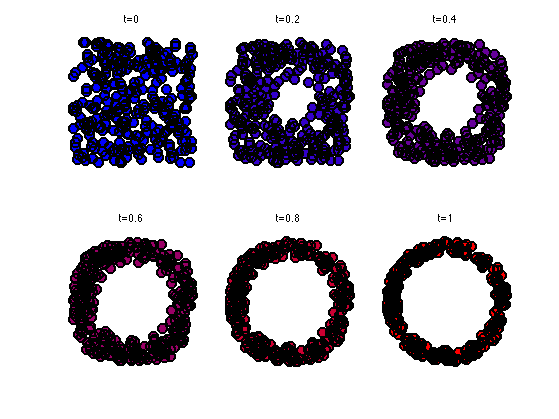## 3D Histogram Matching

We now use this method to perform the equalization of color images, hence $$d=3$$.

Width $$n$$ of the images and number of pixels $$N=n \times n$$.

n = 128;
N = n*n;
d = 3;


Load two color images $$F, G \in \RR^{n \times n \times 3}$$.

F = rescale( load_image('hibiscus', n) );
G = rescale( load_image('flowers', n) );


Display them.

clf;
imageplot({F G});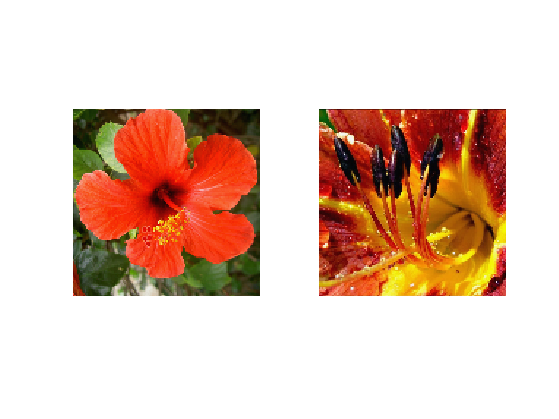The 3-D points clouds $$f,g \in \RR^{N \times 3}$$ associated with the images.

f = reshape(F, [n*n 3])';
g = reshape(G, [n*n 3])';


Shortcut to compute 2-D histograms.

quantize = @(A,Q)1+round((Q-1)*A);
J = @(I,Q)I(1,:)' + Q*(I(2,:)'-1);
hist2d = @(f,Q)reshape( accumarray(J(quantize(f,Q),Q), ones(1,N), [Q*Q 1], @sum), [Q Q]);


Number of bins for the display of histograms.

Q = 60;


Display the 2-D histograms of the red/green channels of both images (use log to boost contrast).

func = @(a)log(a+3);
clf;
imageplot({ func(hist2d(f(1:2,:),Q)), func(hist2d(g(1:2,:),Q)) });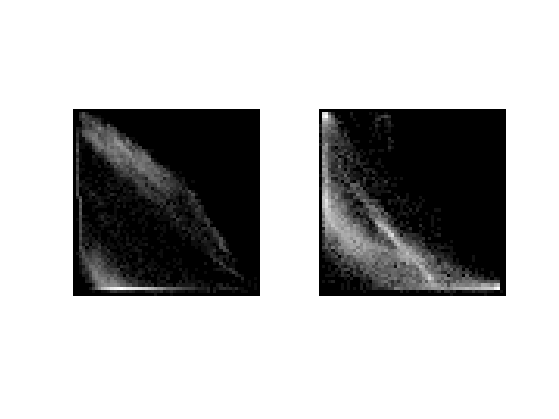Exercice 3: (check the solution) Perform the equalization of each of the coordinate independantly of $$f$$ with $$g$$. Display the resulting image.

exo3;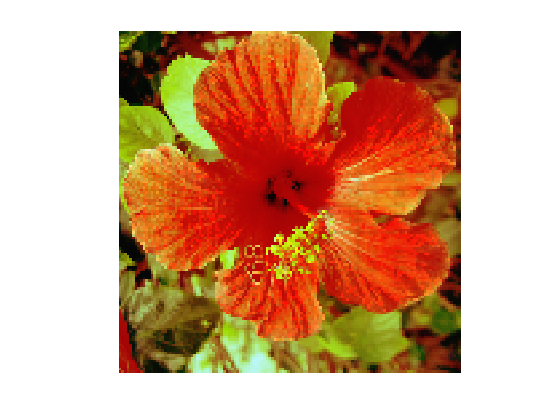Equalizing channel by channel does not leads to an image whose distribution is equal to the target distribution (only the projection on the three axes are equal).

Exercice 4: (check the solution) To obtain an exact matching, one can use the stochastic gradient descent algorithm to minimize $$SW$$. Display the resulting image at several stages of the optimization process.

exo4;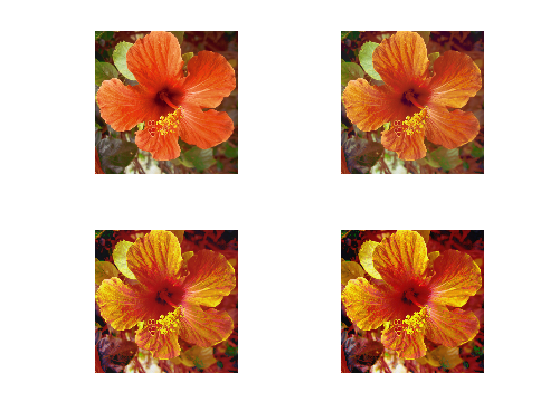Display the final image.

clf;
imageplot(F1);Exercice 5: (check the solution) Display the geodesic interpolation between the two histograms $$\mu_f$$ and $$\mu_g$$.

exo5;